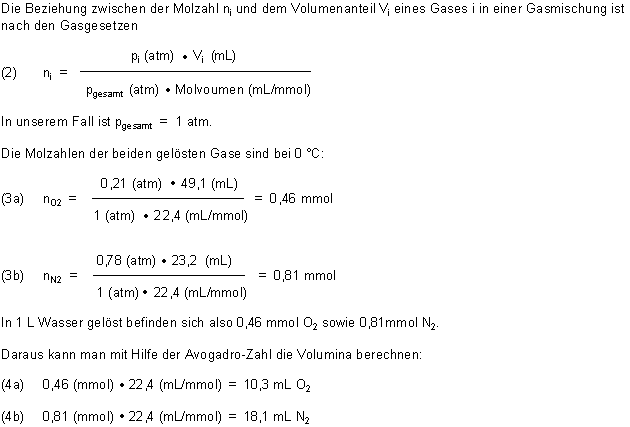# Can dissolve air in water

On the website on the solubility of air in water, we reported on the differences between oxygen and nitrogen.

Above all, it is astonishing that oxygen and nitrogen are present in the air in a volume ratio of O: N = 1: 4, but oxygen is highly enriched in water. Here the volume ratio of the dissolved gases O: N is 1: 1.8. Exactly: At 0 C, 10.3 ml O can be dissolved in 1 liter of water2 and 18.1 ml of N2.

Because of the many inquiries, we derive these values ​​once. But be careful: the whole thing is a bit complicated ...

That’s what’s behind it Henry-Dalton's law: The solubility of a gas at a given temperature is proportional to its pressure. In the case of gas mixtures, the solubility is proportional to the partial pressures of the gases (ideally assumed).

In the following it means to pay close attention: Because we have to differentiate between pure gases and gas mixtures.

Pure gases:
Dissolve in 1 liter of water at 0 C

(1a) oxygen: VO2 = 49.1 cm3

(1b) nitrogen: VN2 = 23.2 cm3

You can find out by chemical analysis.

Gas mixture (clean air):
The partial pressures pi of the two gases correspond to their proportion in the air: 0.21 atm (O2) or 0.78 atm (N.2). (The missing 0.1 atm relates to the noble gases.)In water, the volume ratio of the dissolved gases O: N is actually 1: 1.8.

Further texts on the subject of `` water ''